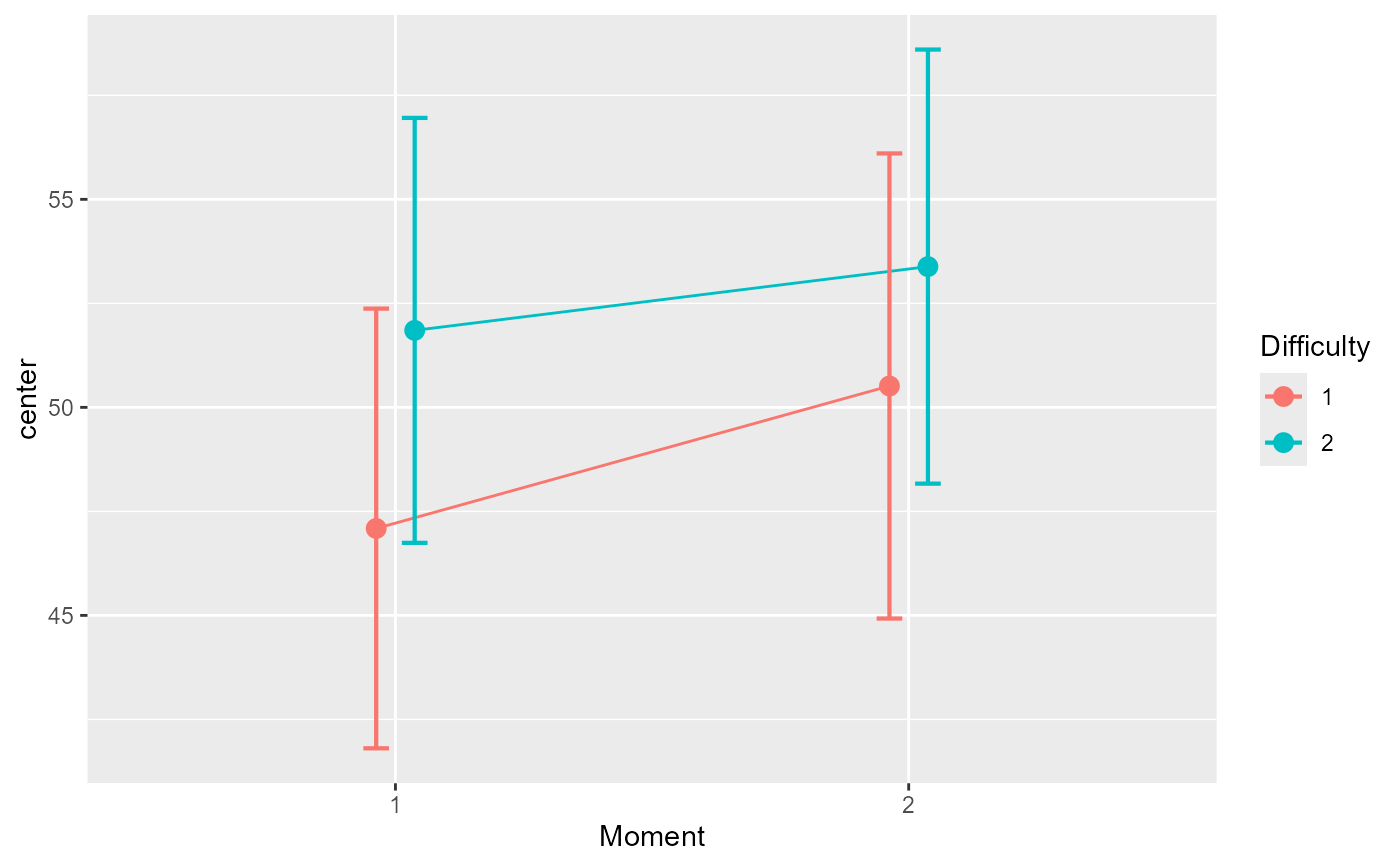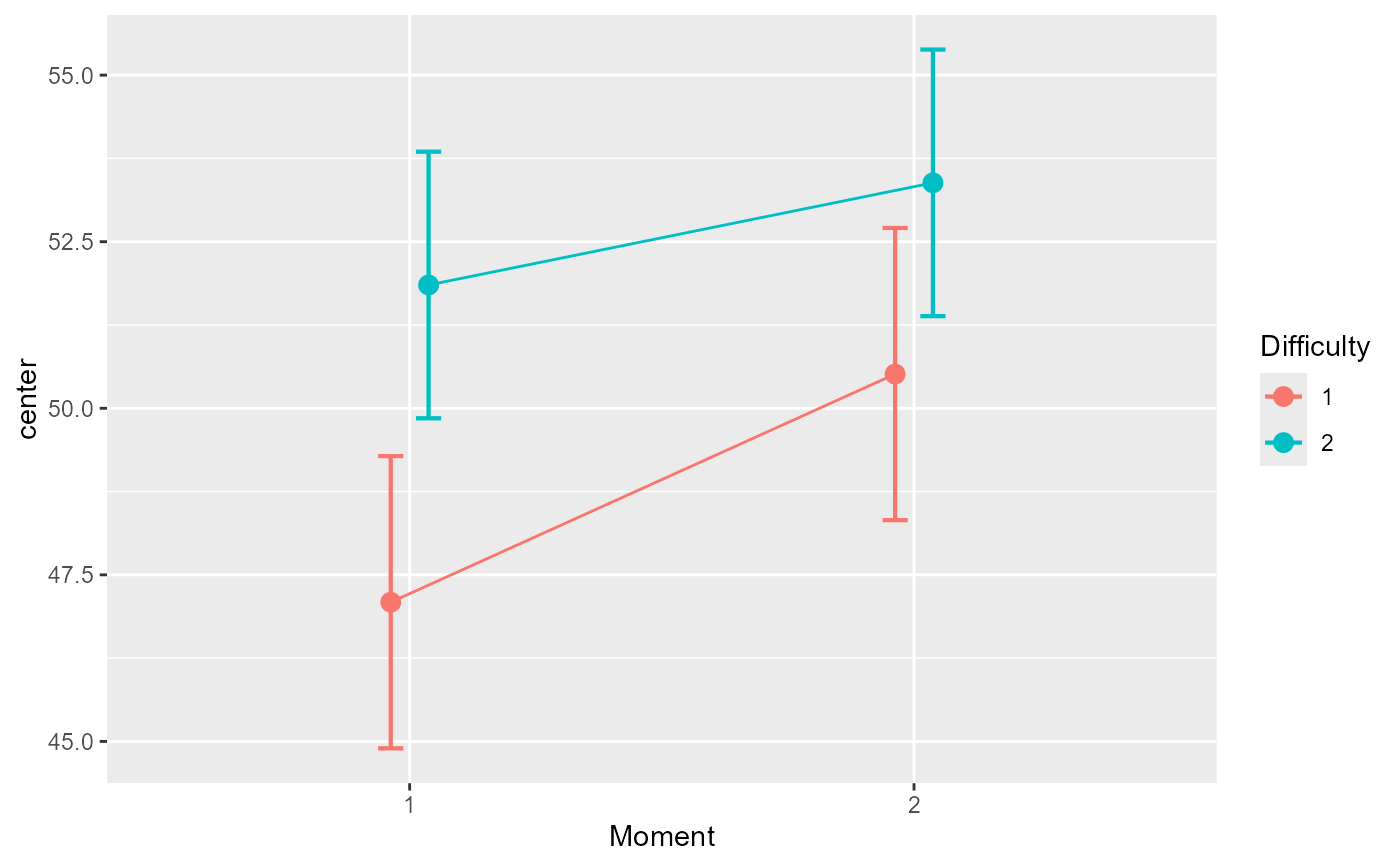The function GRD() generates a data frame containing random data suitable for analyses. The data can be from within-subject or between-group designs. Within-subject designs are in wide format. The function was originally presented in Calderini and Harding (2019) .

GRD(
RenameDV = "DV",
SubjectsPerGroup = 100,
BSFactors = "",
WSFactors = "",
Effects = list(),
Population = list(mean = 0, stddev = 1, rho = 0, scores =
"rnorm(1, mean = GM, sd = STDDEV)"),
Contaminant = list(mean = 0, stddev = 1, rho = 0, scores =
"rnorm(1, mean = CGM, sd = CSTDDEV)", proportion = 0)
)

## Arguments

RenameDV provide a name for the dependent variable (default DV) indicates the number of simulated scores per group (default 100 in each group) a string indicating the between-subject factor(s) with, between parenthesis, the number of levels or the list of level names. Multiple factors are separated with a colon ":" or enumerated in a vector of strings. a string indicating the within-subject factor(s) in the same format as the between-subject factors a list detailing the effects to apply to the data a list providing the population characteristics (default is a normal distribution with a mean of 0 and standard deviation of 1) a list providing the contaminant characteristics and the proportion of contaminant (default 0)

## Value

a data.frame with the simulated scores.

## Note

Note that the range effect specification has been renamed extent to avoid masking the base function base::range.

Calderini M, Harding B (2019). “GRD for R: An intuitive tool for generating random data in R.” The Quantitative Methods for Psychology, 15(1), 1--11. doi: 10.20982/tqmp.15.1.p001 .

## Examples

 # Simplest example using all the default arguments:
dta <- GRD()
#>   id         DV
#> 1  1 -0.9286630
#> 2  2  1.2211696
#> 3  3 -0.4366338
#> 4  4  0.7789042
#> 5  5  0.0532047
#> 6  6  0.4404194
hist(dta$DV)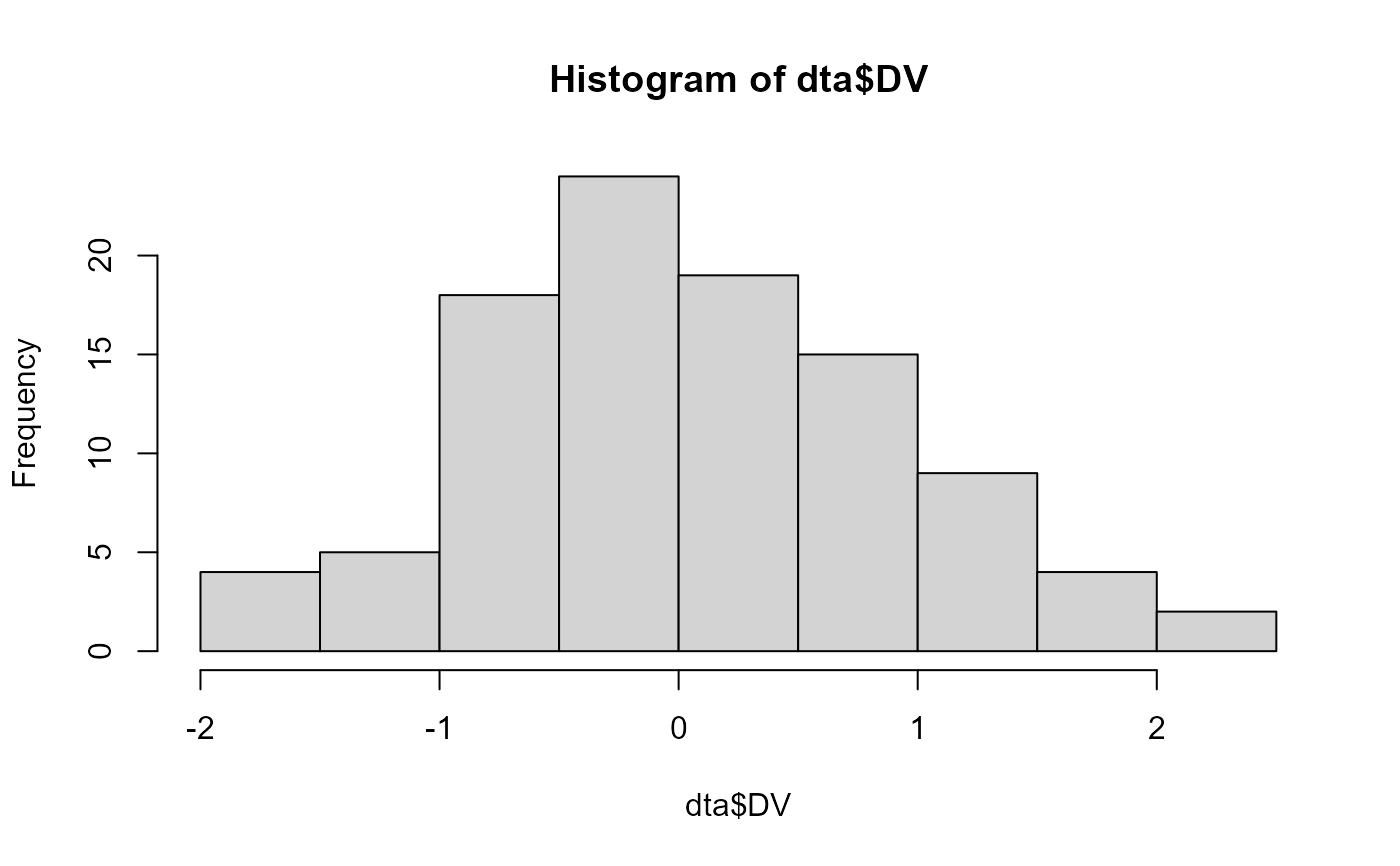# Renaming the dependant variable and setting the group size: dta <- GRD( RenameDV = "score", SubjectsPerGroup = 1000 ) hist(dta$score )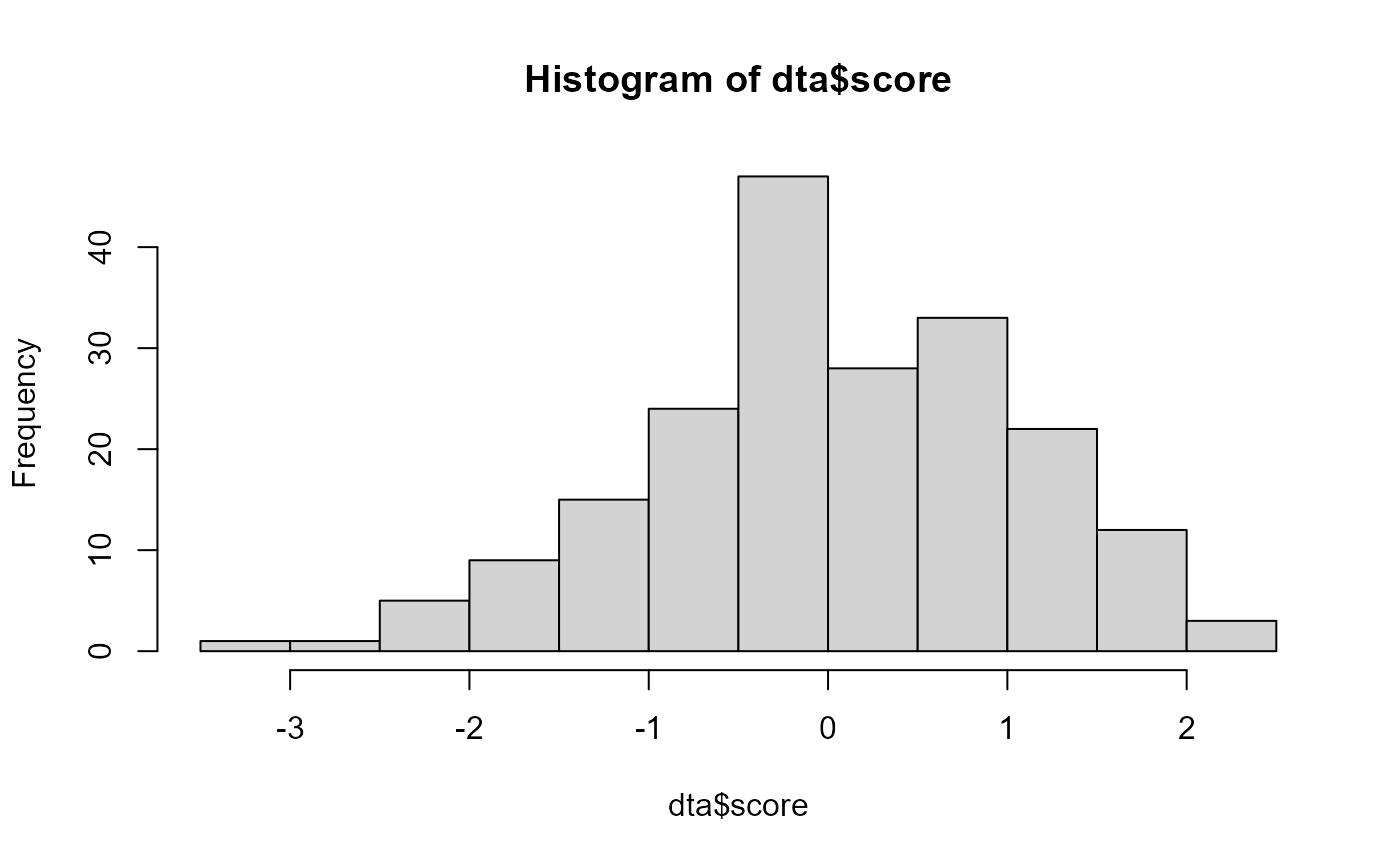# Examples for a between-subject design and for a within-subject design:
dta <- GRD( BSFactors = '3')
dta <- GRD( WSFactors = "Moment (2)")

# A complex, 3 x 2 x (2) mixed design with a variable amount of participants in the 6 groups:
dta <- GRD(BSFactors = "difficulty(3) : gender (2)",
WSFactors="day(2)",
SubjectsPerGroup=c(20,24,12,13,28,29)
)

# Defining population characteristics :
dta <- GRD(
RenameDV = "IQ",
Population=list(
mean=100,  # will set GM to 100
stddev=15  # will set STDDEV to 15
)
)
hist(dta\$IQ)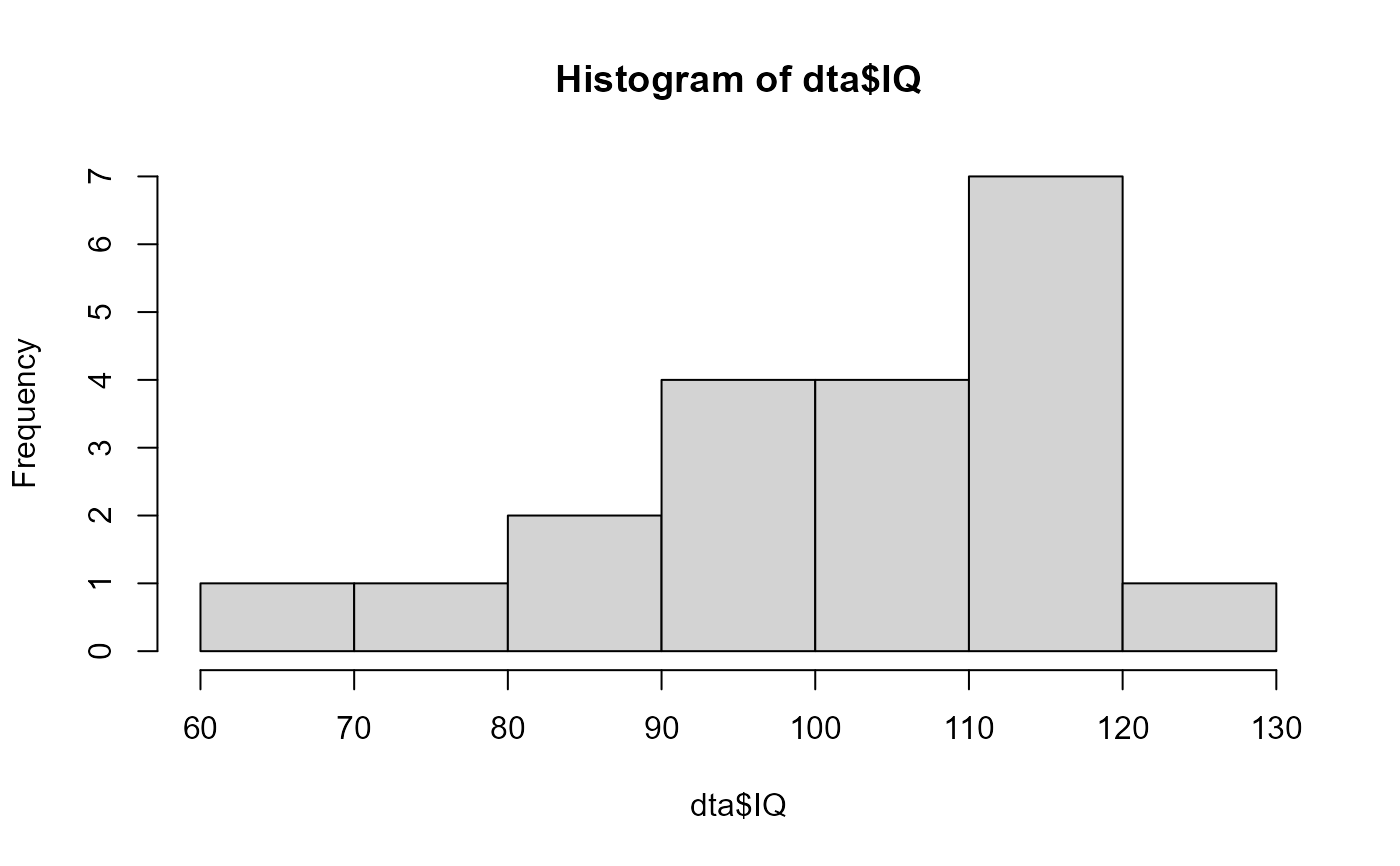# This example adds an effect along the "Difficulty" factor with a slope of 15
dta <- GRD(BSFactors="Difficulty(5)", SubjectsPerGroup = 100,
Population=list(mean=50,stddev=5),
Effects = list("Difficulty" = slope(15) )  )
# show the mean performance as a function of difficulty:
superbPlot(dta, BSFactors = "Difficulty", variables="DV")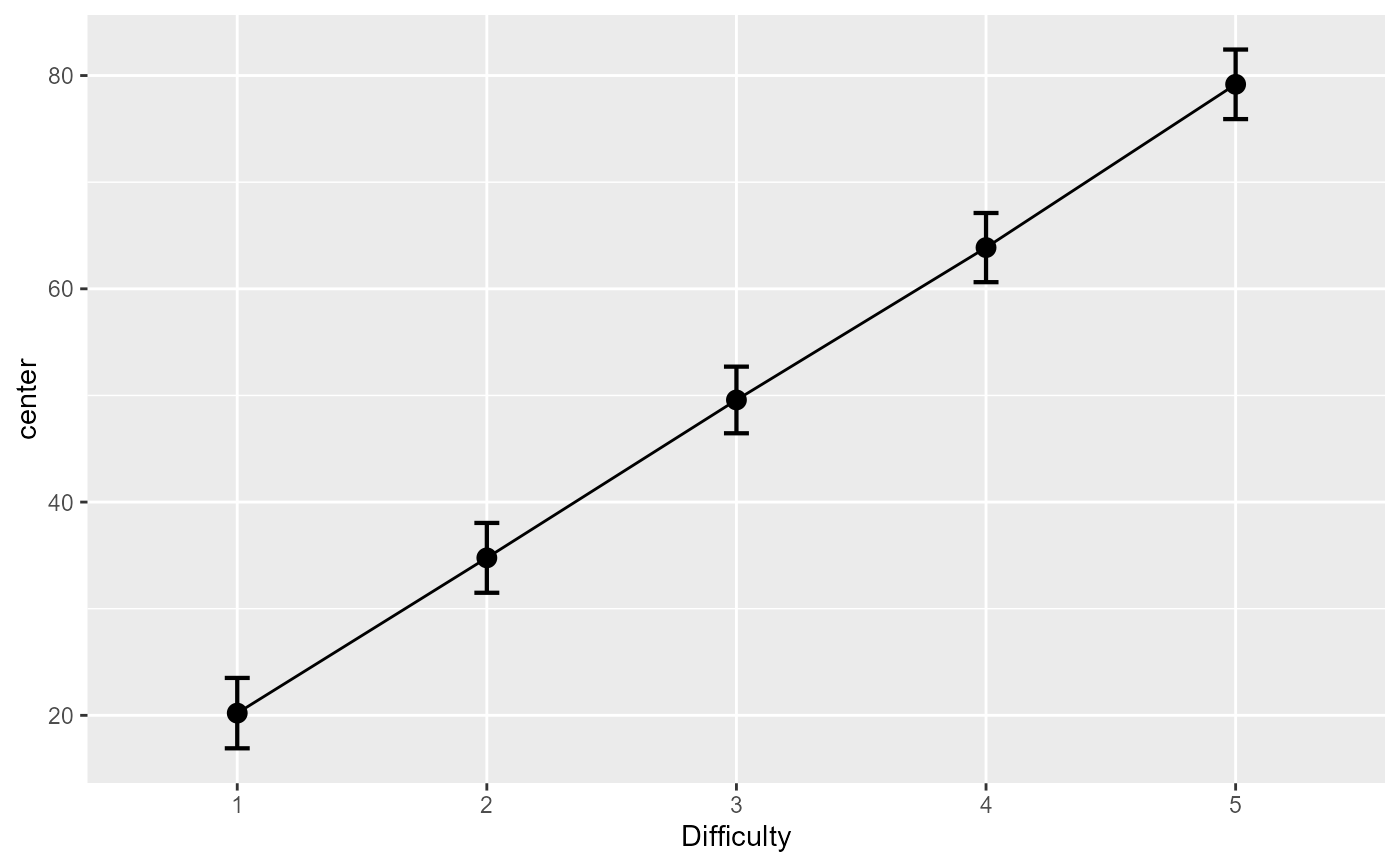# An example in which the moments are correlated
dta <- GRD( BSFactors = "Difficulty(2)",WSFactors = "Moment (2)",
SubjectsPerGroup = 1000,
Effects = list("Difficulty" = slope(3), "Moment" = slope(1) ),
Population=list(mean=50,stddev=20,rho=0.85)
)
# the mean plot on the raw data...
superbPlot(dta, BSFactors = "Difficulty", WSFactors = "Moment(2)",
variables=c("DV.1","DV.2"), plotStyle="line",